# Algorithmic worlds

## -

Back to the information page

Introduction
Algorithmic art
Pictorial algorithms
Ultra Fractal
Algorithmic worlds
Piling patterns
The structure
Pattern generators
Index operators
The piling operator
An example
Other modules

## Truchet patterns

The idea behind Truchet pattern is fairly simple. Start from a regular tiling of the plane (most often a square tiling), as well as a set of tile decorations. Then apply to each tile a random decoration. Because of the random choice, the underlying regularity of the tiling is broken, what yields interesting patterns.

Truchet's original decorations consist in dividing the square into two triangles along a diagonal and paint them with different colors (see the Wikipedia article). It is more interesting to devise the decorations so that they merge smoothly at the edges of the tiles, hiding the underlying tiling.

My works based on Truchet patterns can be found here. Let us describe now a few examples.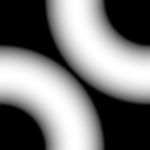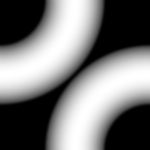Two decorations of square tiles...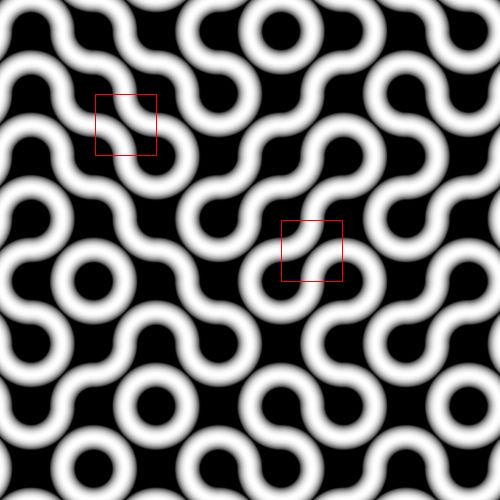...and the Truchet pattern constructed by applying them randomly to a square tiling. Two elementary squares carrying the two types of decorations are highlighted in red.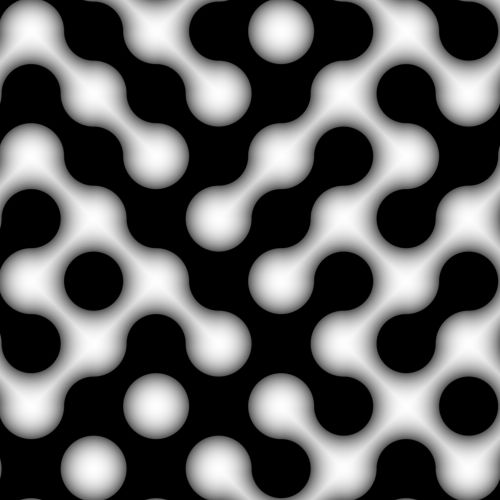Here, two different sets of decorations are used on two subsets of the square tiling. Those correspond to the tiles colored black and white on a checker.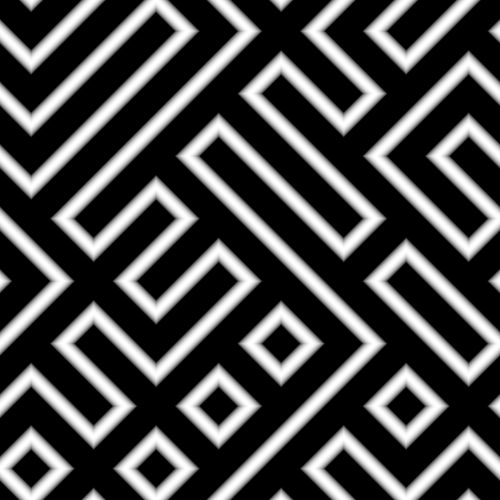In this case, the decorations are similar as in the first example, except that the circle arcs are replaced by straight diagonal lines.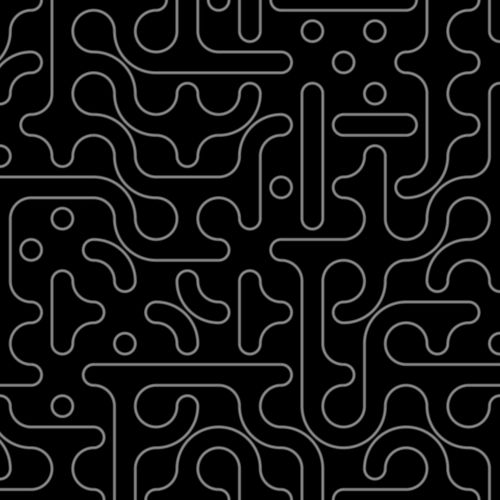In this case, there are a larger number of decorations. Like in the first example, each decoration is composed of non-intersecting "strings" drawn on the squares. While in the first example, one string was crossing each edge of the squares, here two strings do.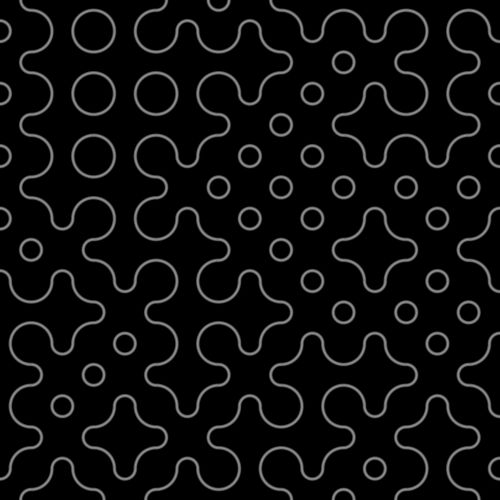This Truchet pattern is constructed from only two of the decorations used in the previous example.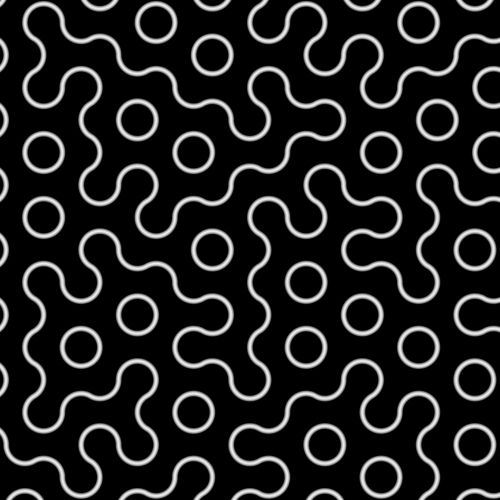Here, a hexagonal tiling has been used, instead of a square one.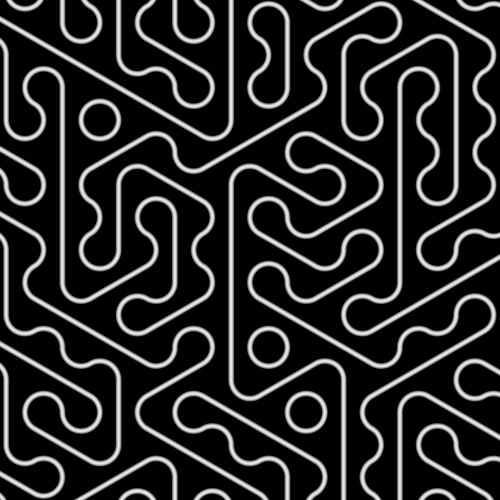A variation also based on an hexagonal tiling.

 Copyright S.Monnier 2009-2019. Bookmark or share